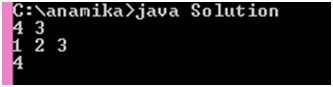# Coin change problem and solution in Java

Here, we are going to solve a problem of called Coin change problem using java programming. This problem can be solved by using dynamic programming.
Submitted by Anamika Gupta, on June 01, 2018

Problem:

You are working at the cash counter at a fun-fair, and you have different types of coins available to you in infinite quantities. The value of each coin is already given. Can you determine the number of ways of making change for a particular number of units using the given types of coins?

Example:

If you have 3 types of coins, and the value of each type is given as 1,2,3 respectively, you can make change for 4 units in four ways:

```    {1,1,1,1}
{1,1,2}
{1,3}
{2,2}

Distribute as total sum is 4.

f(4)=1+f(3)
f(4)=2+f(2)
f(4)=3+f(1)
f(3)=1+f(2) and so on
```

Solution code (java code):

```import java.io.*;
import java.util.*;
import java.text.*;
import java.math.*;
import java.util.regex.*;

public class Solution {
static long[][]  memo;
static long count(int i,long coin[],int j){
if(i==0)
return 1;
if(i<0)
return 0;
if(j<=0)
return 0;
if(memo[i][j]!=-1)
return memo[i][j];
memo[i][j]=count(i,coin,j-1)+count(i-(int)coin[j-1],coin,j);
//memo[i][j]=memo[i][j-1]+memo[i-value(c[j-1])][j]
//where  0<=j<=m hence j-1 is used.
//for example if c[]=1,2,3 and n=4 then
//memo=memo+memo[4- value(c])]
//i.e. memo=memo+memo
return memo[i][j];
}

public static void main(String[] args) {
Scanner in = new Scanner(System.in);
int n = in.nextInt();
int m = in.nextInt();
long c[]=new long[m];

memo=new long[n+1][m+1];

for(int i=0;i<=n;i++)
for(int j=0;j<=m;j++)
memo[i][j]=-1;
for(int c_i=0; c_i < m; c_i++){
c[c_i] = in.nextLong();
}
long ways=count(n,c,m);
// Print the number of ways of making change for 'n'
//units using coins having the values given by 'c'
System.out.println(ways);
}
}
```

OutputWhat's New (MCQs)

Top Interview Coding Problems/Challenges!

Languages: » C » C++ » C++ STL » Java » Data Structure » C#.Net » Android » Kotlin » SQL
Web Technologies: » PHP » Python » JavaScript » CSS » Ajax » Node.js » Web programming/HTML
Solved programs: » C » C++ » DS » Java » C#
Aptitude que. & ans.: » C » C++ » Java » DBMS
Interview que. & ans.: » C » Embedded C » Java » SEO » HR
CS Subjects: » CS Basics » O.S. » Networks » DBMS » Embedded Systems » Cloud Computing
» Machine learning » CS Organizations » Linux » DOS
More: » Articles » Puzzles » News/Updates

© https://www.includehelp.com some rights reserved.